# Test: Electrical Machines- 1

## 10 Questions MCQ Test GATE Electrical Engineering (EE) 2023 Mock Test Series | Test: Electrical Machines- 1

Description
Attempt Test: Electrical Machines- 1 | 10 questions in 30 minutes | Mock test for Electrical Engineering (EE) preparation | Free important questions MCQ to study GATE Electrical Engineering (EE) 2023 Mock Test Series for Electrical Engineering (EE) Exam | Download free PDF with solutions
QUESTION: 1

### A 50 KVA, 11000/400 V Δ-Y distribution transformer has a resistance of 1% and reactance of 7% per unit. What is the transformer phase impedance referred to the high-voltage side?

Solution:

∴ Its base impedance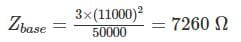The PU impedance of the transformer is
Zeq = [0.01 + j 0.07] PU
So the high-side impedance in ohm is
Zeq = Zeq pu × Zbase
= (0.01 + j0.07) × 7260 Ω
= 72.6 + j 508.2

*Answer can only contain numeric values
QUESTION: 2

### An auto transformer is used to connect a 12.6 kV distribution line to a 13.8 kV distribution line. It must be capable of handling 2000 kVA. There are three phases, connected Y-Y with their neutrals solidly grounded. What must the NC/NSE­ turn ratio be to accomplish this connection? (NC→ common turn, NSE → Series turn)

Solution:

The transformer is connected y-y so the primary and secondary phase voltage are the line voltage divided by 3–√3. The turn ratio of each auto transformer is given by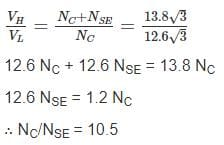QUESTION: 3

### A 3-phase bank of three single phase transformer are fed from 3 phase 33 kV (L-L). It supplies a load of 5000 kVA at 11 kV (L-L). Both supply and load three wire. Calculate the voltage rating of single phase transformer for start-delta connection.

Solution:

For star-delta connection
Primary side phase voltage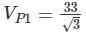Secondary side phase voltage = 11
∴ Transformer voltage rating 19.05/11 kV

QUESTION: 4

Three single phase transformers are connected to form a3 - ϕ transformer bank the transformer are connected in the following manner.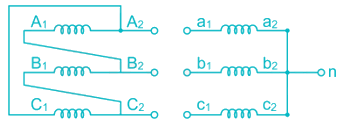the transformer connecting will be represented by

Solution:

Phase diagram of primary and secondary voltage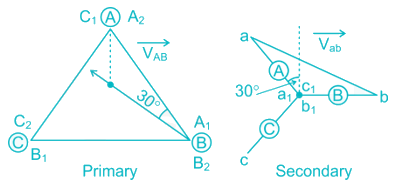this connection is known as Dy11 connectiong

*Answer can only contain numeric values
QUESTION: 5

A 100,000-KVA 230/115 – kV Δ-Δ three phase power transformer has a per-unit resistance of 0.02 PU and a per unit reactance of 0.055. The excitation branch element are RC = 110 PU and XM = 20 PU. If this transformer supplies a load of 80 MVA at 0.85 P.F lagging. Find the voltage regulation (in %) of the transformer bank under these conditions.

Solution:

The transformer supplies a load 80 MVA at 0.85 P.F lagging.

∴ Secondary line current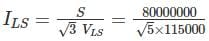= 402 A

The base value of the secondary line current is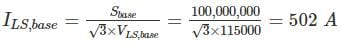So, the P.U secondary current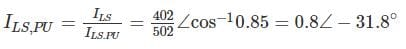The per unit primary voltage of this transformer
VP = VS + IZEQ = 1∠0° +0.8∠ − 31.8° (0.02 + j0.55)
= 1.037 ∠1.6°
The voltage regulation 1.037−11×100 = 3.7%

*Answer can only contain numeric values
QUESTION: 6

The terminal voltage on the secondary side when supplying full load at 0.8 lagging P.F. is 440 V. the equivalent Resistance and reactance drops for the transformer are 1% and 5% respectively.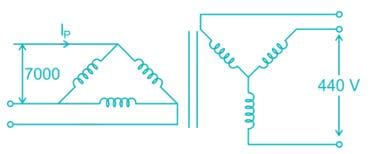Find Induced voltage per phase (in volt) at the secondary side.

Solution:

Percentage voltage Regulation
= R cos ϕ + X sin ϕ
= 1 × 0.8 + 5 × 0.6 = 3.8%
We know that voltage regulation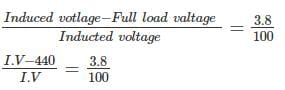I.V. – 440 = 0.038 I.V
I.V = 457.38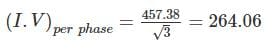*Answer can only contain numeric values
QUESTION: 7

A1 ϕ 33 kVA, 3300 V/330 V, 50 Hz, distribution transformer is to be connected as an auto-transformer to get an output voltage of 3630 V. It maximum kVA rating as an auto-transformer is________kVA

Solution: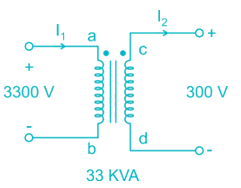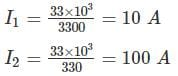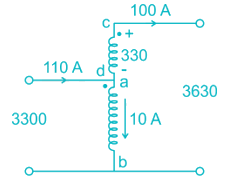Maximum kVA rating = V.A = VHIH = 3630 × 100 = 363 KVA

*Answer can only contain numeric values
QUESTION: 8

A 50 Hz, 3ϕ core type star-delta transformer has a line valtage ratio of 11,000/440 volts. The cross-section of the core is square with a circumscribing circle of 0.5 m diameter. If maximum flux density of 1.30 ωb/m2 then find the number of turns per phase on high voltage windings. Assume insulation occupies 8% of the total core area.

Solution:

We known that diameter of the circumscribing is same as diagonal of the square.
Area of square core = l × b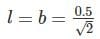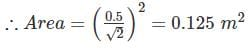∴ Net cross section area = 0.125 × 0.92 = 0.115
E.M.F/Turn = 4.44 fBA = 4.44 × 50 × 1.3 × 0.115 = 33.19
Phase turn ratio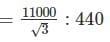∴ Number of turn per phase on high voltage side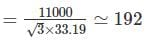QUESTION: 9

In a Star\Delta 3 phase transformer connection, the turns ratio is N1/N2 with N1 being primary side turns and N2 being secondary side turns. What is the value of line voltage and current on secondary side, if the same for primary side are V & I respectively ?

Solution:

line voltage at primary = V
Phase voltage at primary  =V/√3
Line current at primary = I
Phase current at primary = I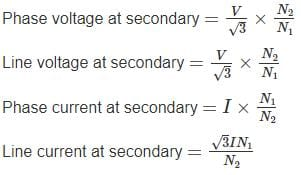QUESTION: 10

A 3 – phase, Δ – Y, 33000 / 660 V, 150 kVA, 50Hz transformer is having resistance per phase of 4 Ω on primary side and 0.15 Ω on secondary side. If the efficiency of transformer and half load is 96.5% at 0.85 pf (lagging), then what are the iron losses?

Solution: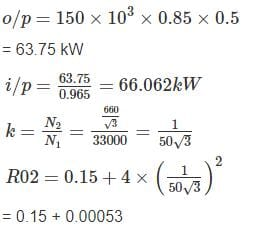= 0.15053 Ω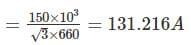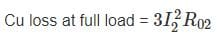= 3 × (131.216)2 × 0.15053
= 7,775.31 W
Cu loss at half load = (0.5)2 × 7775.31
= 1943.83 W
Losses = input - output
= 66062 – 63750
= 2312
Iron loss = total loss – cu loss
= 2312 – 1943.83
= 368.17 WUse Code STAYHOME200 and get INR 200 additional OFF Use Coupon Code